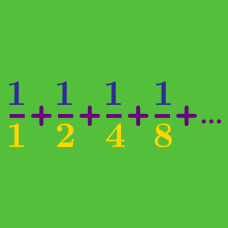Calculus

# Sequences and Series - Problem Solving

$\frac11, \frac21, \frac12, \frac31, \frac22, \frac13, \frac41, \frac32, \frac23, \frac14, \cdots$

If the $n^\text{th}$ term of the above sequence is $\frac{3}{15}$, what is $n$?

If $\{a_n\}$ is a geometric progression where all terms are positive and $a_{3}=\frac{1}{4}$ and $a_{7}=\frac{1}{64},$ what is the smallest integer $n$ such that $a_n <\frac{1}{1000}?$

Sequence $\{a_n\}$ satisfies $a_1=3, a_{n+1}=\frac{n}{n+1} a_n,$ where $n$ is a positive integer. What is the value of $a_{133}$?

Sequence $\{a_n\}$ satisfies $a_2=2a_1, a_{n+2}-4a_{n+1}+3a_n=0,$ where $n$ is a positive integer. If $a_5=287$, what is the value of $a_4$?

A sequence $\{a_n\}$ with $a_n>0$ for all $n(\ge 1)$ satisfies

$\frac{a_{n+1} - a_n}{a_{n+1} + a_n} = \frac12.$

If $a_1 = 2$, what is $a_5$?

×